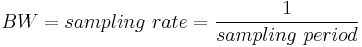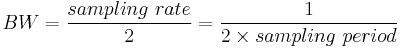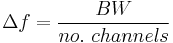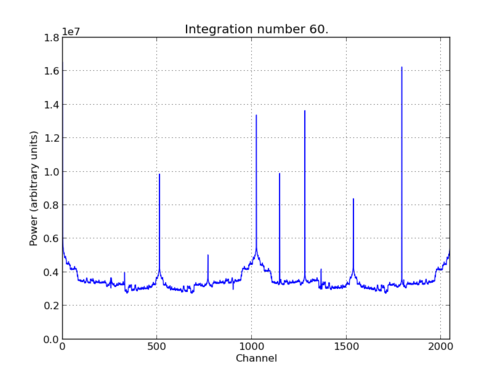# Tutorial 3: Wideband Spectrometer¶

## Introduction¶

A spectrometer is something that takes a signal in the time domain and converts it to the frequency domain. In digital systems, this is generally achieved by utilising the FFT (Fast Fourier Transform) algorithm. However, with a little bit more effort, the signal to noise performance can be increased greatly by using a Polyphase Filter Bank (PFB) based approach.

When designing a spectrometer for astronomical applications, it’s important to consider the science case behind it. For example, pulsar timing searches will need a spectrometer which can dump spectra on short timescales, so the rate of change of the spectra can be observed. In contrast, a deep field HI survey will accumulate multiple spectra to increase the signal to noise ratio. It’s also important to note that “bigger isn’t always better”; the higher your spectral and time resolution are, the more data your computer (and scientist on the other end) will have to deal with. For now, let’s skip the science case and familiarize ourselves with an example spectrometer.

## Setup¶

This tutorial comes with a completed model file, a compiled bitstream, ready for execution on ROACH, as well as a Python script to configure the ROACH and make plots. Here

## Spectrometer Basics¶

When designing a spectrometer there are a few main parameters of note:

• Bandwidth: The width of your frequency spectrum, in Hz. This depends on the sampling rate; for complex sampled data this is equivalent to:In contrast, for real or Nyquist sampled data the rate is half this:as two samples are required to reconstruct a given waveform .

• Frequency resolution: The frequency resolution of a spectrometer, Δf, is given by,

and is the width of each frequency bin. Correspondingly, Δf is a measure of how precise you can measure a frequency.

• Time resolution: Time resolution is simply the spectral dump rate of your instrument. We generally accumulate multiple spectra to average out noise; the more accumulations we do, the lower the time resolution. For looking at short timescale events, such as pulsar bursts, higher time resolution is necessary; conversely, if we want to look at a weak HI signal, a long accumulation time is required, so time resolution is less important.

## Configuration and Control¶

### Hardware Configuration¶

The tutorial comes with a pre-compiled fpg file, which is generated from the model you just went through (snap_tut_spec.fpg) Load up your SNAP. You don’t need to telnet in to the SNAP; all communication and configuration will be done by the python control script called snap_tut_spec.py.

Next, you need to set up your SNAP. Switch it on, making sure that:

• You have your 10MHz reference clock connected to the appropriate SNAP input.

### The snap_tut_spec.py spectrometer script¶

Once you’ve got that done, it’s time to run the script. If you’re in linux, browse to where the snap_tut_spec.py file is in a terminal and at the prompt type

``` ./snap_tut_spec.py <SNAP IP or hostname> -b <fpgfile name>
```

replacing with the IP address of your SNAP and with your fpgfile. You should see a spectrum like this:In the plot, there should be a fixed DC offset spike; and if you’re putting in a tone, you should also see a spike at the correct input frequency. If you’d like to take a closer look, click the icon that is below your plot and third from the right, then select a section you’d like to zoom in to. The digital gain (-g option) is set to maximum (0xffff_ffff) by default to observe the ADC noise floor. Reduce the gain (decrease the value (for a -10dBm input 0x100)) when you are feeding the ADC with a tone, as not to saturate the spectrum.

Now you’ve seen the python script running, let’s go under the hood and have a look at how the FPGA is programmed and how data is interrogated. To stop the python script running, go back to the terminal and press ctrl + c a few times.

### iPython walkthrough¶

The tut3.py script has quite a few lines of code, which you might find daunting at first. Fear not though, it’s all pretty easy. To whet your whistle, let’s start off by operating the spectrometer through iPython. Open up a terminal and type:

```ipython
```

and press enter. You’ll be transported into the magical world of iPython, where we can do our scripting line by line, similar to MATLAB. Our first command will be to import the python packages we’re going to use:

```import corr,time,numpy,struct,sys,logging,pylab
```

Next, we set a few variables:

```katcp_port = 7147
```

Which we can then use in FpgaClient() such that we can connect to the ROACH and issue commands to the FPGA:

```fpga = casperfpga.CasperFpga(snap)
```

We now have an fpga object to play around with. To check if you managed to connect to your ROACH, type:

```fpga.is_connected()
```

Let’s set the bitstream running using the progdev() command:

```fpga.upload_to_ram_and_program('snap_tut_spec.fpg')
```

Now we need to configure the accumulation length and gain by writing values to their registers. For two seconds and maximum gain: accumulation length, 2*(2^28)/2048, or just under 2 seconds:

```fpga.write_int('acc_len',2*(2**28)/2048)
fpga.write_int('gain',0xffffffff)
```

Finally, we reset the counters:

```fpga.write_int('cnt_rst',1)
fpga.write_int('cnt_rst',0)
```

```acc_n = fpga.read_uint('acc_cnt')
```

Do this a few times, waiting a few seconds in between. You should be able to see this slowly rising. Now we’re ready to plot a spectrum. We want to grab the even and odd registers of our PFB:

```a_0=struct.unpack('>1024l',fpga.read('even',1024*4,0))
```

These need to be interleaved, so we can plot the spectrum. We can use a for loop to do this:

```interleave_a=[]

for i in range(1024):
interleave_a.append(a_0[i])
interleave_a.append(a_1[i])
```

This gives us a 2048 channel spectrum. Finally, we can plot the spectrum using pyLab:

```pylab.figure(num=1,figsize=(10,10))
pylab.plot(interleave_a)
pylab.title('Integration number %i.'%acc_n)
pylab.ylabel('Power (arbitrary units)')
pylab.grid()
pylab.xlabel('Channel')
pylab.xlim(0,2048)
pylab.show()
```

Voila! You have successfully controlled the SNAP spectrometer using python, and plotted a spectrum. Bravo! You should now have enough of an idea of what’s going on to tackle the python script. Type exit() to quit ipython. snap_tut_spec.py notes ==

Now you’re ready to have a closer look at the snap_tut_spec.py script. Open it with your favorite editor. Again, line by line is the only way to fully understand it, but to give you a head start, here’s a few notes:

Connecting to the SNAP

To make a connection to the SNAP, we need to know what port to connect to, and the IP address or hostname of our SNAP. The connection is made on line 73:

```fpga = casperfpga.CasperFpga(snap)
```

The katcp_port variable is set on line 13, and the roach variable is passed to the script at the terminal (remember that you typed python snap_tut_spec.py roachname). We can check if the connection worked by using fpga.is_connected(), which returns true or false:

```if fpga.is_connected():
```

The next step is to get the right bitstream programmed onto the FPGA fabric. The bitstream is set on line 68, from the options your entered on cli:

```bitstream = opts.fpgfile
```

Then the progdev command is issued on line 108:

```fpga.upload_to_ram_and_program(bitstream)
```

Passing variables to the script

Starting from line 51, you’ll see the following code:

```from optparse import OptionParser

p = OptionParser()
p.set_usage('spectrometer.py <ROACH_HOSTNAME_or_IP> [options]')
p.set_description(__doc__)
help='Set the number of vectors to accumulate between dumps. default is 2*(2^28)/2048, or just under 2 seconds.')
help='Skip reprogramming the FPGA and configuring EQ.')
help='Specify the fpg file to load')
opts, args = p.parse_args(sys.argv[1:])

if args==[]:
print 'Please specify a SNAP board. Run with the -h flag to see all options.\nExiting.'
exit()
else:
snap = args
if opts.fpgfile != '':
bitstream = opts.fpgfile
```

What this code does is set up some defaults parameters which we can pass to the script from the command line. If the flags aren’t present, it will default to the values set here.

## Conclusion¶

If you have followed this tutorial faithfully, you should now know:

• What a spectrometer is and what the important parameters for astronomy are.

• Which CASPER blocks you might want to use to make a spectrometer, and how to connect them up in Simulink.

• How to connect to and control a SNAP spectrometer using python scripting.

In the following tutorials, you will learn to build a correlator, and a polyphase filtering spectrometer using an FPGA in conjunction with a Graphics Processing Unit (GPU).